What voltage is needed to create a current of 43 mA in a circuit containing only a 1.3-μF capacitor, when the frequency is 3.9 kHz? Note: Th

Question

What voltage is needed to create a current of 43 mA in a circuit containing only a 1.3-μF capacitor, when the frequency is 3.9 kHz? Note: The ac current and voltage are rms values and power is an average value unless indicated otherwise.

in progress 0
5 months 2021-08-19T09:05:16+00:00 1 Answers 0 views 0

1.35 V

Explanation:

Given:

Capacitance of the capacitor (C) = 1.3 μF =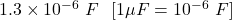Frequency (f) = 3.9 kHz= 3.9 × 1000 Hz = 3900 Hz [1 kHz = 1000 Hz]

Current flowing in the circuit (I) = 43 mA = 0.043 A [1 mA = 0.001 A]

Now, as only capacitor is there in the circuit, the impedance of the circuit is equal to the reactance of the capacitor.

So,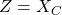The reactance of the capacitor is given as: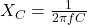Plug in the values given and solve for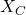. This gives,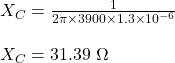Therefore, the impedance is,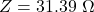Now, the voltage needed to create the given current is obtained using the formula from Ohm’s law and is given as: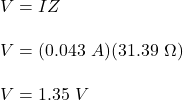Therefore, the voltage needed to create a current of 43 mA is 1.35 V.